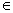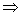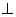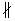# Mapping of a Plane with Motion Graphing

• ### Корзина

ПустоThe contents of this webpage:

• – the lesson "Geometric mapping of planes" is presented in the example of solving problems 139 - 141;
• – in the worksheets under numbers 142 - 143 of this workbook for mathematics, the motion in geometry is considered;
• – the lesson "Equal rectangles" is explained in the example of the problem 144.

## Geometric mapping of planes

The axial symmetry and the central symmetry are examples of the geometric mapping of the plane.

When a plane is mapped onto itself:

1) every point of the plane is put in correspondence with the unique point of the plane.

2) each point of the plane is placed in correspondence with some point of the plane.

Definition:

The motion is a map of the plane onto itself such that the distance between the points is kept.

Examples: the point A lies on the line L,

the point B does not lie on the line La) draw the point A relative to the line L:

A → A (see Figure a)

Draw the point B relative to the line L:

B → B1

1) the segment BB1 is perpendicular to the line L2) the segments OB = OB1 are equal (see Figure a)

b) draw the points A, B and the segment AB relative to the line L (see Figure b):

A → A1

B → B1

AB → A1B1c) draw the segment AB relative to the line L (see Figure c):

the segments are parallel: AB || A1B1d) draw the segment AB relative to the point O (see Figure d):

A → A1 : AO = A1O

B → B1 : BO = B1O

***

Problem 139.

Given:

L – is the axis of the symmetry,

the straight line a is parallel to the line L

a || L

a1 – is the mapping of aa → a1

Prove:

the straight line a1 is parallel to the line L

a1 || L

Proof:

Let us take any two points A and B on the line a. We obtain the segment ABa, lying on the line a. Find the mapping of the segment AB. To get that, we draw perpendiculars to the axis L from the points B and A.

Having measured the distance from the points A or B to the axis L, we postpone the same distance by a compass, i.e.A → A1

B → B1

We connect the point A1 with the point B1 and obtain the segment A1B1a1, lying on the line a1.

It follows, by the construction:

AO = OA1, BO1 = O1B1

Since AB || L , we see that AO = BO1 = O1B1 = OA1

Then OA1 = OB1, i.e. the points A1 and B1 are at an equal distance from the axis L.

Therefore, the line a1 is parallel to the line L

a1 || L

***

Problem 140.

Given:

a is the axis of the symmetry, the line

Build:

a figure F is a map of the given quadrilateral

Construction:

1) draw perpendiculars from points A, B, C, D to the axis a.2) measure the distance with the help of a compass from the point O to the points A and D and postpone them so that the distance between the points A and O, D and O is equal to the distance between the symmetrical distances D1O and A1O.

3) Measure the distance with the help of the compass from the point O1 to the points B and C and postpone them so that the distance between the points B and O1, C and O1 is equal to the distance between the symmetrical distances B1O1 and C1O1.

4) Connect in series the points A1, B1, C1, D1.

By construction, the figure F is the quadrilateral.

***

Problem 141.

Given:

L is the axis of the symmetry

the straight line a is perpendicular to the line L

aLProve:

the straight line a is mapped onto itself relative to the axis L

aa

Proof:

1) any pointAa, lying on the line a, is placed in correspondence with the point A1a, also lying on the line a (by the definition of the symmetry relative to the line L; aL ).

2) any pointA1a, lying on the line a, is placed in correspondence with the point Aa, lying on the line a.

Therefore, the line a is mapped onto itself relative to the axis L

aa

***

## Motion

Theorem:

When moving, the segment is mapped to the segment.

Given:

MN – is the segment

MM1

NN1Prove:

the segment MN is mapped to the segment M1N1

MNM1N1

Proof:

1) We take a point PMN, lying on the segment MN.

Then MP + PN = MN (*)

By moving the point P is mapped to the point P1

PP1

Since the motion keeps the distance between the points, then

MN = M1N1; MP = M1P1, PN = P1N1 (1)

Then it follows from equalities (*) and (1) that

M1P1 + P1N1 = M1N1 , therefore the point P1 lies on the segment M1N1

P1M1N1

Then the segment MN is mapped to the segment M1N1

MNM1N1

2) We verify the second condition.

Any pointP1M1N1, lying on the segment M1N1, gives the equality M1P1 + P1N1 = M1N1 (**)

By moving the point P1 is translated to the point P

P1P

It follows from (**) and (1) that MP + PN = MN

Therefore, the point PMN lies on the segment MN.

So, by movement, the segment MN is translated into the segment M1N1:

MNM1N1

***

Consequence 1:

The triangle is translated to a triangle equal to it.

Given:

the triangle ΔABC

AA1

BB1

CC1

Prove:

the triangle ΔABC is translated into the equal triangle ΔA1B1C1

ΔABCΔA1B1C1: ΔABC = ΔA1B1C1

Proof:

By the proved theorem, the segment is translated into the equal segment.

Then

1) by the motion, the segment AB is translated into its equal segment A1B1 , i.e. ABA1B1 , so that AB = A1B1

2) by the motion, the segment AC is translated into its equal segment A1C1 , i.e. ACA1C1 , so that AC = A1C1

3) by the motion, the segment BC is translated into its equal segment B1C1 , i.e. BCB1C1 , so that BC = B1C1

Therefore, by the motion, the triangle ΔABC is translated into an equal triangle ΔA1B1C1, i.e.

ΔABCΔA1B1C1:

ΔABC = ΔA1B1C1 (according to three sides)

***

Consequence 2:

The straight line is translated into a straight line, the ray is translated into a ray, the angle is translated into an equal angle.

Problem 142.

Given:

two straight lines are parallel a || b

aa1

bb1

Prove:

parallelism of the lines, i.e. two straight lines are parallel a1 || b1

Suppose that two lines are not parallel a1b1, then these lines intersect a1b1

Each point of the lines a and b corresponds to a unique point of the lines a1 and b1.

Each point of the lines a1 and b1 corresponds to the point of the straight lines a and b.

Then the point of the intersection of the lines a1 and b1 corresponds to the intersection point of the lines a and b, but this claim contradicts the condition of the problem.

Therefore, the two lines are parallel a1 || b1

***

Problem 143.

Given:

O is the point of the central symmetry

b is the straight line

Build:

the straight line b1 is a map of the line b relative to the point O

bb1

Construction:

1) Take any two points, for example, A and B, on the line b.

2) Draw the ray BO.3) Draw the ray AO.

4) Let us measure the segment AO with the help of the compass and on the continuation of the ray AO let us postpone from the point O the distance equal to the distance between the points A and O.

5) Let us measure the segment BO with the help of the compass and, on the continuation of the ray BO, postpone the distance equal to the distance between the points B and O from the point O.

6) We obtain two equal segments AB = A1B1

7) We draw the line b1 through A1B1, then we obtain a straight line b1 is a map of the line b relative to the point O

bb1

***

Problem 144.

Given:

ABCD is the rectangle

A1B1C1D1 is the rectangle

a) AB = A1B1, BC = B1C1

b) A1B1 = AB

AC = A1C1 are the diagonals

Prove: ABCD = A1B1C1D1

Proof:

a) Let us prove it by contradiction.

Suppose that ABCD ≠ A1B1C1D1

Then AB ≠ A1B1 and BC ≠ B1C1, but this claim contradicts this condition.

Therefore, the rectangles are equal ABCD = A1B1C1D1

***